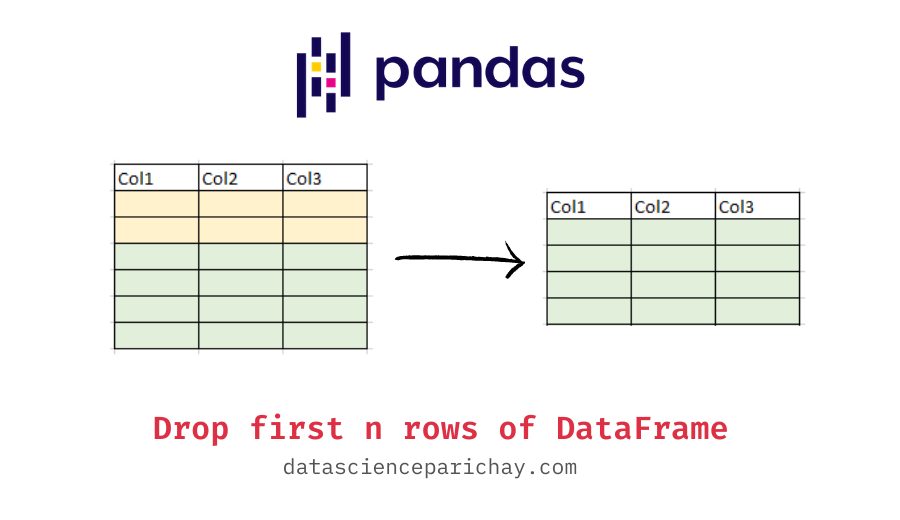# Pandas – Drop first n rows of a DataFrame

In this tutorial, we will look at how to drop the first n rows of a pandas dataframe.

There are a number of ways to remove the first n rows of a dataframe. For example, you can slice the dataframe using `.iloc` or you can use the pandas `drop()` function or you can use the pandas `tail()` function. The following is the syntax for the different methods used in this tutorial.

```# using iloc
df.iloc[n:]
# using drop()
df.drop(df.index[:n])
# using tail()
df.tail(-n)```

Let’s look at some examples of using the above methods to remove the first n rows of a dataframe. First we will create a sample dataframe that we will be using throughout this tutorial.

```import pandas as pd

# create dataframe
df = pd.DataFrame({
'Name': ['Bulbasaur', 'Charmander', 'Squirtle', 'Caterpie', 'Ekans'],
'Type': ['Grass', 'Fire', 'Water', 'Bug', 'Poison']
})
# display the dataframe
print(df)```

Output:

```         Name    Type
0   Bulbasaur   Grass
1  Charmander    Fire
2    Squirtle   Water
3    Caterpie     Bug
4       Ekans  Poison```

We now have a dataframe with five rows containing information on Pokemon names and their respective types.

You can drop the first n rows by slicing them using `.iloc`. For example, let’s remove the first two rows of df and store the resulting dataframe as a separate dataframe.

```# remove top two rows
df_sub = df.iloc[2:]
# display the dataframe
print(df_sub)```

Output:

📚 Data Science Programs By Skill Level

Introductory

Intermediate ⭐⭐⭐

🔎 Find Data Science Programs 👨‍💻 111,889 already enrolled

Disclaimer: Data Science Parichay is reader supported. When you purchase a course through a link on this site, we may earn a small commission at no additional cost to you. Earned commissions help support this website and its team of writers.

```       Name    Type
2  Squirtle   Water
3  Caterpie     Bug
4     Ekans  Poison```

The resulting dataframe has the top two rows removed. Note that, the slice `2:` refers to take all the rows starting from index 2 (that is, the third row). That is why the resulting dataframe has rows from index 2.

You can also use the pandas `drop()` function to remove the first n rows of a dataframe. For this, pass the indexes of the rows you want to delete to the drop() function. For example, let’s again drop the top two rows of the dataframe df, this time using the drop() function.

```# remove top two rows
df_sub = df.drop(df.index[:2])
# display the dataframe
print(df_sub)```

Output:

```       Name    Type
2  Squirtle   Water
3  Caterpie     Bug
4     Ekans  Poison```

The first two rows are dropped in the returned dataframe. If you want to modify the original dataframe in place, pass `inplace=True` to the drop() function.

You can also use the pandas `tail()` function to remove the first n rows. Generally, the tail() function is used to show the last n rows of a pandas dataframe but you can pass a negative value to skip the rows from the beginning.

```# remove top two rows
df_sub = df.tail(-2)
# display the dataframe
print(df_sub)```

Output:

```       Name    Type
2  Squirtle   Water
3  Caterpie     Bug
4     Ekans  Poison```

You can see that the resulting dataframe has all the rows from the bottom except for the first two rows. This is because we passed -2 to the tail() function which equates to performing `df[2:]`.

For more on the pandas tail() function, refer to its documentation.

With this, we come to the end of this tutorial. The code examples and results presented in this tutorial have been implemented in a Jupyter Notebook with a python (version 3.8.3) kernel having pandas version 1.0.5

•